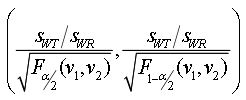## SAS and R (?) for variability comparison (FDA NTID Guidance) [General Sta­tis­tics]

Dear all,
notation clarification for a newbie,
using EMA's sample data set I -full replicate- after removing the subject that didn't complete all periods
regarding this post : ddubins post
for calculation of CV-intra in full replicate design using FDA SAS code provided in appendix E
FDA Guidance
according to ddubins entry about Covariate Parameter Estimates:
Residual Subject Treatment A 0.124 <---- (sig_WT^2, the within-subject standard deviation) for the Test product Residual Subject Treatment B 0.242 <---- (sig_WR^2, the within-subject standard deviation for the Reference product)
however when using the code given in fda guidance for warfrain sodium and following the same idea here to Calculate the 90% confidence interval of the ratio of the within subject standard
deviation of test product to reference product σWT/σWR by using the residual terms in covariates estiames to calculate the upper bound as follows:guidance for warfrain sodium
and calculating the SWr for the critical bound ,the numbers aren't same ,
Covariance Parameter Estimates Cov Parm Subject Group Estimate FA(1,1) Subject   0.8454 FA(2,1) Subject   0.8232 FA(2,2) Subject   0.02062 Residual Subject Formula R 0.2097 Residual Subject Formula T 0.1202 
and Swr obtained from proc mixed step -same idea as Shuanghe- there's slight differenceCovParm  Estimate s2wr  theta      y       boundy sWR     critbound Residual 0.4080 0.20401 1.11006 -0.22647 -0.17419 0.45168 -0.14657 
my first question is and -sorry for the silly long post,the residual terms in covariates estimates is an estimate of the variance for both Test and reference and not the standard deviation as ddubins said?
and that means the calculation will be as follows :
sqrt(Residual Subject Formula R)
and same for Test to obtain the variability ratio?

2nd question,

Regarding Helmut lecture about Reference Scaled Average Bioequivalence (part II: NTIDs)
RSABE NTIDs
using the data set provided: CNS drug data set
when using the fda code for NTID mentioned above in warfarin guidance i get the following values:
Cov Parm Subject Group Estimate FA(1,1) Subject   0.2473 FA(2,1) Subject   0.2680 FA(2,2) Subject   0 Residual Subject Formula R 0.02483 Residual Subject Formula T 0.003281
and
CVwt=SQRT(EXP(0.003281)-1) --> 5.73% CVwr==SQRT(EXP(0.02483)-1) --> 15.86%
CL: 93.90% - 103.35% CovParm    Estimate   s2wr    theta          y         ]boundy     sWR       critbound Residual   0.03095   0.015474   1.11006   -0.017177   -0.010451   0.12439   -0.009828289
when using these estimates to obtain the upper bound for variability comparison i get : 0.60080159
the number checks out with what's in your lecture regarding EMA values but why there's a difference between FDA values ?Thanks in advance and Best RegardsIng. Helmut Schütz